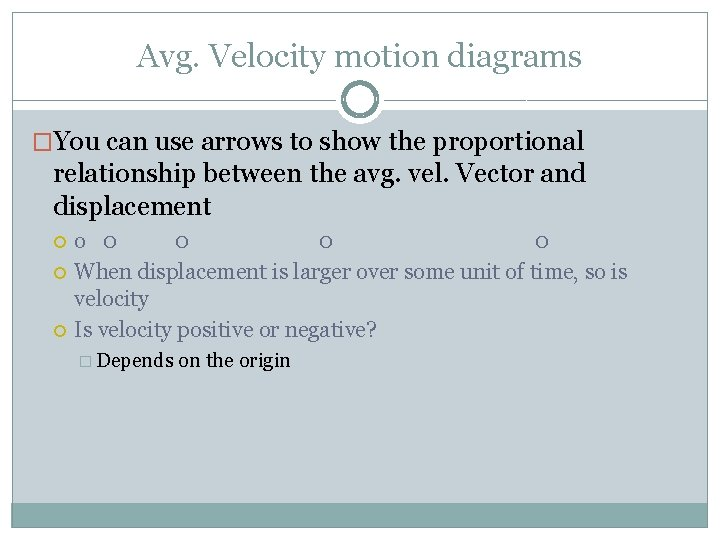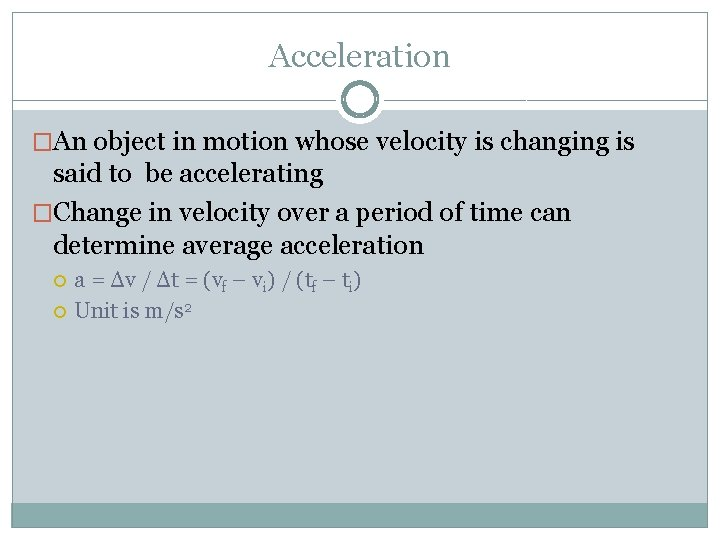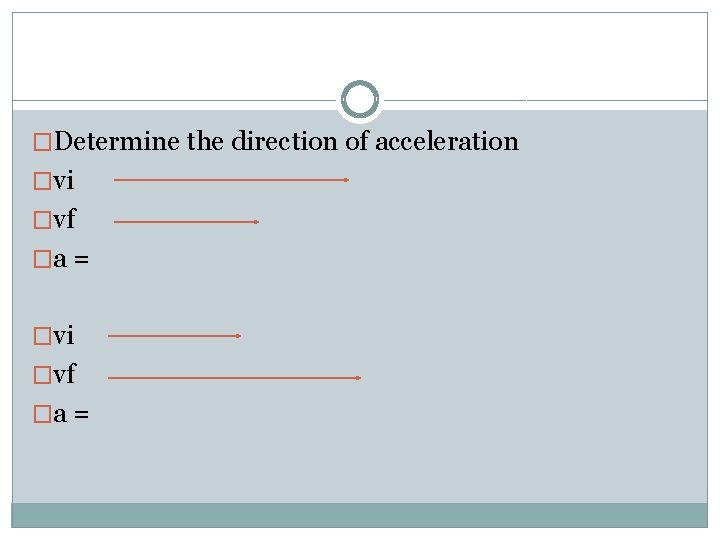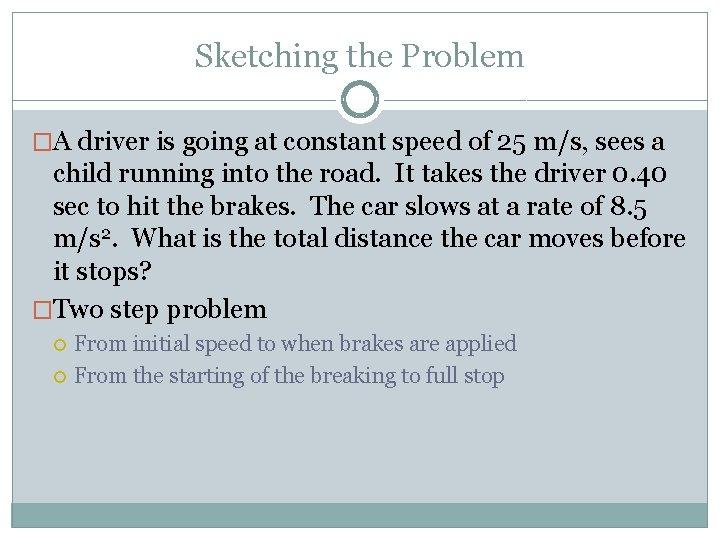# 3 3 Velocity and Acceleration Velocity Average Velocity

• Slides: 11
Download presentation3 -3 Velocity and AccelerationVelocity �Average Velocity Vector measurement that is the change in distance per some change in time V = Δd / Δt = (d 1 – d 0) / (t 1 – t 0) What is the average velocity of a car starting from rest if it travels 400. 0 meters in 11. 3 sec? V = Δd / Δt = (d 1 – d 0) / (t 1 – t 0) (400. 0 m– 0 m) / (11. 3 sec – 0 sec) 35. 4 m/sVelocity �Suppose it takes a sprinter 9. 87 seconds to run a 100. 0 m dash. At half way (50. 0 meters), the sprinter’s time is at 5. 12 sec, determine the average velocity of the sprinter in the last 50. 0 meters.Avg. Velocity motion diagrams �You can use arrows to show the proportional relationship between the avg. vel. Vector and displacement o 0 0 When displacement is larger over some unit of time, so is velocity Is velocity positive or negative? � Depends on the origin�Rearrange the equation by multiplying both sides by Δt Δd = vΔt �Substitute d 1 – d 0 for Δd in first equation d 1 – d 0 = vΔt �Add d 0 to both sides d 1 = d 0 + vΔt� d 1 = d 0 + vΔt �Over some time interval (Δt), the avg. velocity of a moving object results in a change in position equal to vΔtAcceleration �An object in motion whose velocity is changing is said to be accelerating �Change in velocity over a period of time can determine average acceleration a = Δv / Δt = (vf – vi) / (tf – ti) Unit is m/s 2Acceleration �A runner has an initial pace of 5. 60 m/s. In a matter of 3. 00 sec, the runner has a pace of 6. 70 m/s. What was the average acceleration? �a = Δv / Δt = (vf – vi) / (tf – ti) �a = (6. 70 m/s – 5. 60 m/s) / (3. 00 sec – 0 sec)Motion diagrams to obtain Average Acceleration �Finding the Avg. Accel. using motion diagrams by observing the magnitude of each velocity vector �Acceleration vector is proportional to the change in average velocity vector � 0 0 0 o o o � When Avg. velocity is speeding up in the positive direction, Avg. Accel is positive. When Avg. velocity is slowing down in positive direction, Avg. Accel is negative�Determine the direction of acceleration �vi �vf �a =Sketching the Problem �A driver is going at constant speed of 25 m/s, sees a child running into the road. It takes the driver 0. 40 sec to hit the brakes. The car slows at a rate of 8. 5 m/s 2. What is the total distance the car moves before it stops? �Two step problem From initial speed to when brakes are applied From the starting of the breaking to full stop